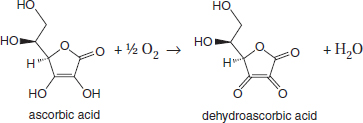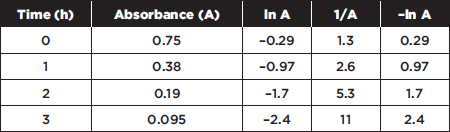# AP Chemistry Practice Test 31

### Test Information13 questions19 minutes

1. Carbon has an atomic radius of 77 pm and a first ionization energy of 1086 kJ/mol.

Based on periodic trends and the data given above, what are the most probable values for the ionization energy and atomic radius of nitrogen?

2. Dissolving one mole of each of the following compounds in water results in solutions with different pH values. Under those conditions, which of the following acids will have the highest percentage ionization?

3.

 Compound Formula Normal Boiling Point, K n-heptane C7H16 371 n-octane C8H18 398 n-nonane C9H20 424 n-decane C10H22 447

Based on the data in the table above, which of the following substances has lowest viscosity?

4. Based on periodic relationships, the concepts related to bond strength, and the concept relating bond strength to acid strength, which of the following correctly predicts the strength of binary acids from strongest to weakest?

5. Chlorine is often used to oxidize other substances. It makes a good bleach because it can oxidize many colored compounds. If chlorine is not available, what other substance can be used for oxidizing substances?

6.Vitamin C is oxidized slowly to dehydroascorbic acid by the oxygen in air. It is catalyzed by ions such as Cu2+ and Fe3+. The reaction can be followed by measuring the ultraviolet absorbance at 243 nm.Which of the following is the best interpretation of the data given above?

7. A new compound is synthesized and found to be a monoprotic acid with a molar mass of 248 g/mol. When 0.0050 mol of this acid are dissolved in 0.500 L of water, the pH is measured as 3.89. What is the pKa of this acid?

8. Which of the following particulate diagrams best represents the reaction of gaseous sulfur (black dots) with oxygen (white circles) to form sulfur dioxide?

9. Nitrous acid is a weak acid, while nitric acid is a much stronger acid because

10. A student has a liter of a 0.100 M solution of a strong acid. To prepare a buffer, this should be mixed with

11.

 Photoelectron Spectroscopy Data Table for Sodium Binding Energy (MJ) Relative Number of Electrons 0.50 1 3.67 6 6.84 2 104 2

The photoelectron data for sodium is shown above. Which of the following statements is true about this data?

12. Identify the Brønsted-Lowry conjugate acid-base pair in the following list.

13. Chemical reactions can be classified as either heterogeneous or homogeneous. Which of the following equations below is best classified as a heterogeneous reaction?